gail_massariCommunity Manager
When Responses Are Below the Limit of Detection

By Mark Bailey MarkBailey, Principal Analytical Training Consultant, Education and Training, SAS

To learn about a system or a process, there must be variation. If the character­istics or outcomes never change, then it is impossible to learn anything. We design experiments to provoke a large change in the response in the hope that the analysis will be more informative, both in kind (factor effects) and degree (precision). The determination of the response requires a measurement that is accurate (unbiased) and precise over a useful range. Many physical quantities are bounded by zero, and all measure­ments are limited by noise. When the response is absent or zero, the background signal can be translated in various ways into an upper bound on measurement, or limit of detection (LOD).1 What value should you use in your analysis for a response reported to be below the limit of detection?

There are many intuitive practices for selecting the value for analysis when the response is below this threshold. Some analysts use zero. Other analysts use the LOD itself. Still others split the difference and use half of the LOD. Finally, some analysts regard such a case as indeter­minate and leave the value missing.

These ad hoc approaches unfortunately do not address the central problem but instead introduce bias in any estimates, such as model parameters. A missing value reduces the sample size and, therefore, the power of the analysis, as if nothing is known about the response when, in fact, there is information avail­able. Using zero biases your estimates downward; using the LOD biases your estimates upward. Using half the LOD might average out the bias, if you are optimistic and tend to be lucky. Isn’t there a better way? Isn’t there a rigorous approach based on statistical theory that eliminates this bias and enables you to use all of the data?

The solution is found in an unrelated field of study that has nothing to do with chemistry or any other physical science. Investigators encountered the same problem at the start of survival analysis. In this analysis, the response is the life­time or the time-to-event where the event is death or the onset of disease. Subjects often survive or never incur the disease during the study period. What to do with their data? Ignoring it or using an arbitrary value would introduce bias as described above.

Analysts realized that two types of data existed in these studies: for one kind, the actual lifetime is known, and for the other kind, it is a lower bound on the actual lifetime. The second kind is called censored data. These lifetimes are right-censored because the actual lifetime is greater than the observation. In the same way, the responses that are below the LOD are called left-censored data.

Ordinary least squares regression is not able to analyze censored responses, but maximum likelihood estimation accommodates censoring directly through the likelihood function.

JMP provides a parametric analysis of survival models with multiple factors, which suits the case of our experiment. The normal distribution is not available for the likelihood function in the Parametric Survival platform, but the log-normal distribution is available. We merely transform the response by exponentiating the response as ey for the analysis and then transform back using the natural logarithm after fitting the model for prediction. The following fictitious example illustrates the points above and shows how to use JMP for such an analysis.

Limit of Detection Example

This example illustrates censoring with responses below the LOD. The experi­ment includes four continuous factors, X1-X4. The response Y is simulated without censoring, and then an arbitrary LOD is applied. Perhaps Y is the level of a chemical impurity that you intend to minimize through judicious selection of factor levels. An arbitrary LOD (10) was selected to cause a few responses to become censored. A thorough study of this matter would involve many simulated data sets. The single simulated set of responses here is presented only to illustrate the problem and how to deal with it.

The setup for this problem in JMP is simple. The original columns for the experiment are in the left red box in Figure 1.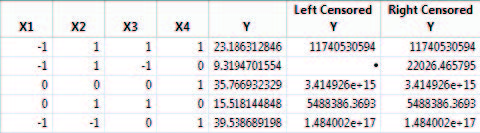Figure 1 Original data (left) and new censored data columns (right)

Three columns were added to facilitate the analysis as seen in the right box in the figure. We use interval censoring for this analysis. That is, we specify a lower and upper bound for each response in two new columns, here called Left Censored Y and Right Censored Y, respectively. These values are the same as the exponentiated actual response when it is above the LOD as seen in the first row. The left-censored value is missing and the right-censored value is the exponentiated LOD for censored responses as seen in the second row.

We will examine the bias caused by using one of the ad hoc corrections before examining the results of using the correct analysis.

The data were fit to a second order polynomial function with all two-factor interaction terms using ordinary least squares regression. The value in column Y was the simulated response levels before imposing the LOD. This regres­sion represents the best we could do if there was no limit of detection. It is our benchmark for comparison with different ways of handling a LOD.

The OLS regression analysis is repeated with three different versions of the response Y.

1. The value in column Y2 was set to 0 if the simulated response was below the LOD.

2. The value in column Y3 was set to halfway between 0 and LOD (5 in this case) if the simulated response was below the LOD.

3. The value in column Y4 was set to the LOD (10 in this case) if the simu­lated response was below the LOD.

The prediction formulas from all four OLS regressions are saved to the data table. The estimates for the parameters that are active in the simulated response are compiled from each of these four regressions along with the true value from the simulation. Figure 2 shows the results in Graph Builder.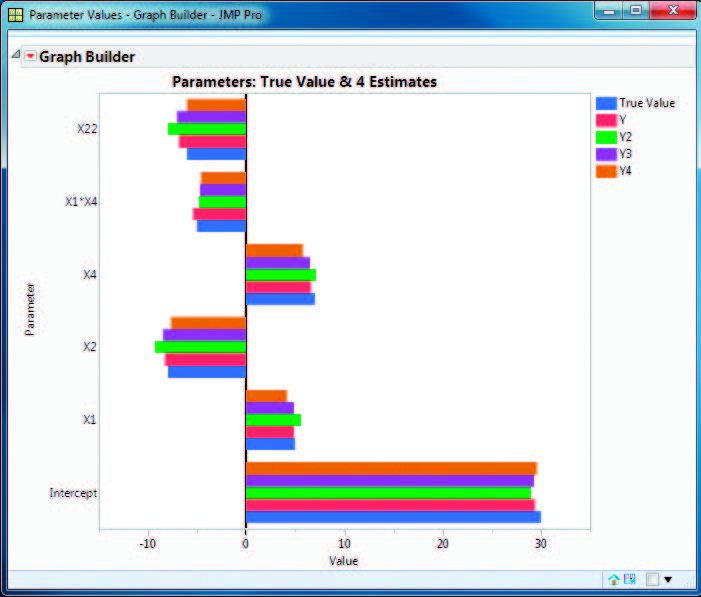Figure 2 Actual versus predicted parameter estimates

Notice that the estimates are close to the true value when there is no LOD (Y). On the other hand, the application of one of the three ad hoc methods when the response is below the LOD results in estimates that are not as close to the true value. (Note that this single example is not sufficient for proof. It is intended only to illustrate the problem.)

Now try the parametric survival model. In Fit Model, change the fitting Personality from Standard Least Squares to Parametric Survival, select the Lognormal for the Distribution, and assign the Left Censored Y and Right Censored Y to the Time to Event role. The terms in this model (Location Effects) are the same as the terms used in the OLS regression above. There are no terms for the Scale Effects. This situation treats the variance as a constant, independent of factor levels.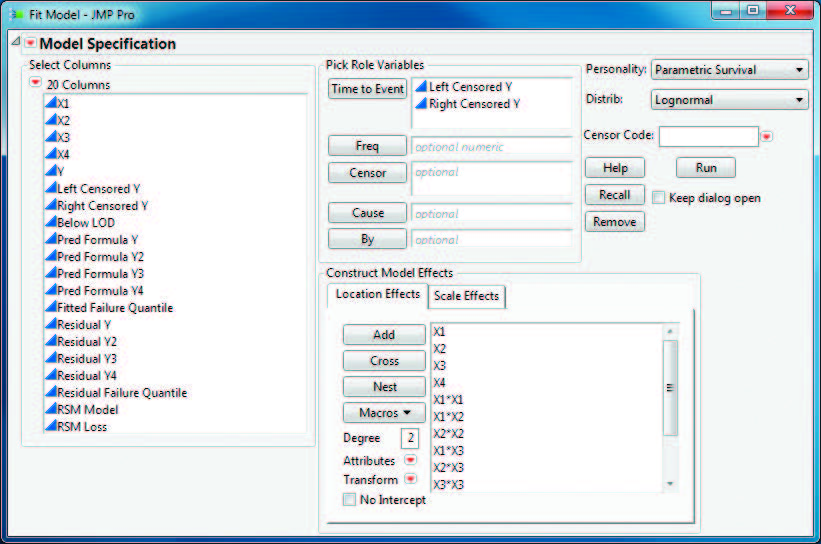Figure 3 Fit Model specification for the Parametric Survival model

The results must be translated as follows. The linear model determines the location parameter of the log-normal distribution and sigma estimates the standard deviation of the same distribu­tion. Save the prediction formula for the time quantile. Click the red triangle at the top of the report, select Save Quantile Function, and then enter 0.5 for the probability. A new column called Fitted Failure Quantile is added to the data table. You can rename this column to reflect the original response.

The last step is to edit the prediction formula to transform back to the original response. This step is easy. When you open the formula editor for the new column, the entire formula is already selected. Simply select the Transcendental group of functions, select Log, and then save the new formula.

Figure 4 shows the first five rows of the updated data table, which includes the original response Y and the new Fitted Failure Quantile formula column. The four new Y columns were created by saving the prediction formula for the four OLS regressions and the parametric survival regression. Notice that the Fitted Failure Quantile prediction is much closer to that from Pred Formula Y, the first OLS regression (our benchmark).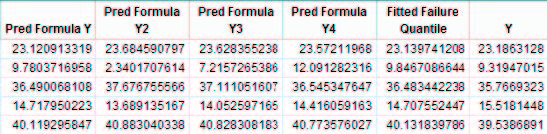Figure 4 Data table with new prediction columns

Examine the correspondence between the observed response and the predicted response from all of the models so far. In Figure 5, a graph of these predicted responses overlays a scatterplot. The identity line (Y=X) was added for reference.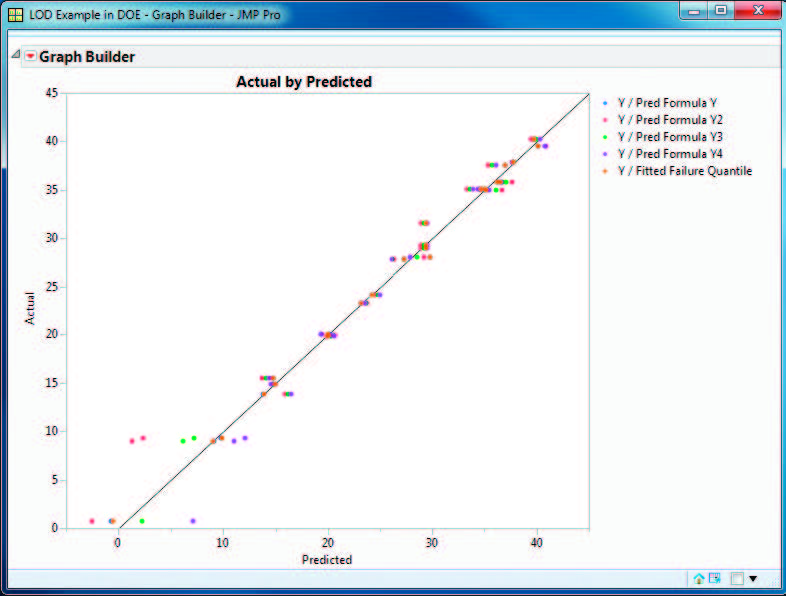Figure 5 Graph of actual by predicted responses

The markers closest to the Y=X line are those from the first OLS regression (no LOD imposed) and the parametric survival regression. The discrepancy becomes worse as the response approaches the LOD.

Perhaps a better way to see the distinctions between these different approaches is with a residual plot (Figure 6).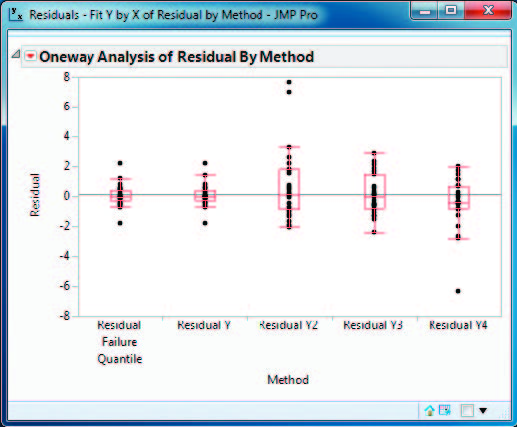Figure 6 Residual plot of responses by method

The residuals closest to 0 are those from the first OLS regression when no LOD was imposed (Residual Y) and the parametric survival regression (Residual Failure Quantile). The large residuals from the models using ad hoc adjust­ments to the response (increased bias) indicate that these models are less valuable if you need predictions near the LOD, as in the case of optimizing factor levels to reduce an impurity.

In conclusion, I have demonstrated that ad hoc methods for using a response below the detection limit result in biased parameter estimates and model predictions. On the other hand, using interval censoring with a parametric survival model avoids these problems. The survival model is easy to fit. The transformations allow the log-normal distribution to easily model the errors. You can use the prediction formula with tools such as the Prediction Profiler to find optimal settings. Further note that you can use the same approach when the limiting response is an upper bound by substituting a missing value for the Right Censored Y value.

Article Labels

There are no labels assigned to this post.

Article Tags